#### Study Material and Notes of Ch 10 Light – Reflection Class 10th Science

Topics in the Chapter

• Introduction
• Properties of Light
• Reflection
→ Laws of Reflection
→ Virtual and Real image
• Image formed by Plane mirror
→ Characteristics of image formed by Plane mirror
→ Lateral inversion and its application
• Spherical Mirrors
→ Properties of Concave mirror
→ Properties of Convex mirror
→ Common terms for Spherical mirrors
• Rules for making ray diagrams by spherical mirrors
• Ray diagrams for images formed by concave mirror
→ When object is at infinity
→ When object is beyond C
→ When object is at C
→ When object is placed between F and C
→ When object is placed at F
→ When object is between P and F
• Uses of Concave mirror
• Ray diagrams of images formed by convex mirror
→ When object is placed at infinity
→ When object is placed between pole and infinity
• Uses of convex mirror
• Sign convention for reflection by spherical mirror
• Mirror Formula
→ Magnification of Spherical mirrors

Introduction

→ Light is the form of energy that provides sensation of vision.

→ Some common phenomena associated with lights are image formation by mirrors, the twinkling of stars, the beautiful colours of a rainbow, bending of light by a medium and so on.

Properties of Light

→  Electromagnetic wave, so does not require any medium to travel.

→  Light tends to travel in straight line.

→  Light has dual nature i.e. wave as well as particle.

→ Speed of light is maximum in vaccum. Its value is 3 × 108 ms-1.

→ When light falls on a surface, following may happen:
(i) Reflection
(ii) Refraction
(iii) Absorption

Reflection

→ Bouncing back of light when it strikes on a polished surface like mirror.

Laws of Reflection

(i) Angle of incidence is equal to the angle of reflection.

(ii) The incident ray, the reflected ray and the normal at the point of incidence, all lie in the same plane.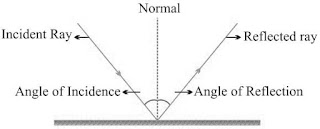Virtual and Real image

Image is a point where atleast two light rays actually meet or appear to meet.

 Real Image Virtual Image Formed when light rays actually meet. Formed when light rays appear to meet. Can be obtained on screen. Can’t be obtained on screen. Inverted Erect Example: image formed on cinema screen and formed by concave mirror. Example: image formed by plane mirror or convex mirror.

Image Formed by Plane MirrorCharacteristics of Image formed by Plane Mirror

(i)  Virtual and erect.
(ii) Size of image is equal to the size of object.
(iii) Image is formed as far behind the mirror as the object is in front of it.
(iv) Laterally inverted.

Lateral Inversion: The right side of the object appears left side of the image and vice-versa.

Application of lateral inversion

→ The word AMBULANCE is written in reverse direction so that it can be read correctly in rear view mirror of vehicles going in front of it.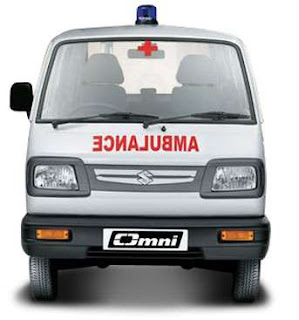Spherical Mirrors

→ Mirrors whose reflecting surface is curved.

→ There are two types of spherical mirrors:
(i) Convex Mirror
(ii) Concave Mirror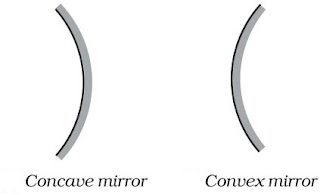Properties of Concave mirror

• Reflecting surface is curved inwards.
• Converging mirror

Properties of Convex mirror

• Reflecting surface is curved outwards.
• Diverging mirror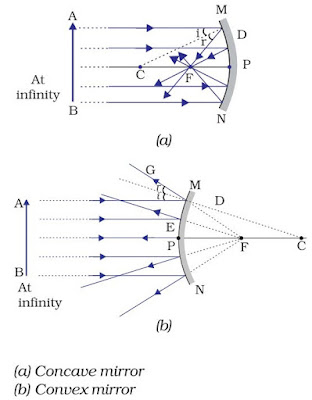Common terms for Spherical mirrors

Principal axis: The line joining the pole and center of curvature.

Pole (P): The centre of the spherical mirror.

Aperture (MN):  It is the effective diameter of the spherical mirror.

Center of Curvature (C): The centre of the hollow glass sphere of which the mirror was a part.

Radius of Curvature (R): The distance between the pole and the centre of curvature.

Focus (F): The point on principal axis where all the parallel light rays actually meet or appear to meet after reflection.

Focal length (f): The distance between the pole and the focus.

→ Relationship between focal length and radius of curvature: f = R/2

Rules for making ray diagrams by spherical mirror

(i)  A ray parallel to the principal axis, after reflection, will pass through the principal focus in case of a concave mirror or appear to diverge from the principal focus in case of a convex mirror.(ii) A ray passing through the principal focus of a concave mirror or a ray which is directed towards the principal focus of a convex mirror, after reflection, will emerge parallel to the principal axis.(iii) A ray passing through the centre of curvature of a concave mirror or directed in the direction of the centre of curvature of a convex mirror, after reflection, is reflected back along the same path.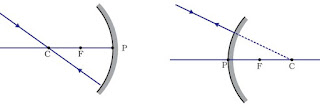(iv) A ray incident obliquely to the principal axis, towards a point P (pole of the mirror), on the concave mirror or a convex mirror, is reflected obliquely. The incident and reflected rays follow the laws of reflection at the point of incidence (point P), making equal angles with the principal axis.Ray diagrams for images formed by concave mirror

(i) When object is at infinity
Image Position − At ‘F’
Nature of image – Real, inverted
Size – Point sized or highly diminished(ii) When object is beyond ‘C’
Image Position – Between ‘F’ and ‘C’
Nature of image – Real, inverted
Size – Diminished(iii) When object is at ‘C’
Image Position – At ‘C’
Nature of image – Real, inverted
Size – Same size as that of object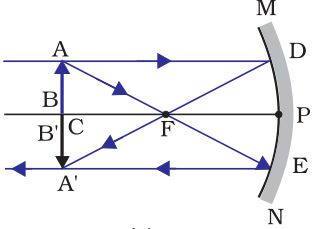(iv) When object is placed between ‘F’ and ‘C’
Image Position – Beyond ‘C’
Nature of image– Real, inverted
Size – Enlarged(v) When object is placed at ‘F’
Image Position – At Infinity
Nature of image – Real, inverted
Size – Highly enlarged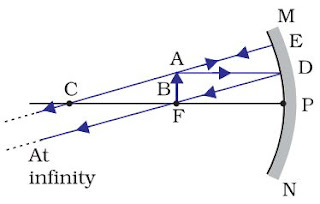(vi) When object is between ‘P’ and ‘F’
Image Position – Behind the mirror
Nature of image – Virtual, erect
Size – EnlargedUses of Concave Mirror

(i) Used in torches, search lights and vehicles headlights to get powerful parallel beam of light.

(ii) Concave mirrors are used by dentists to see large image of teeth of patients. (Teeth have to be placed between pole and focus).

(iii) Concave mirror is used as shaving mirror to see a larger image of the face.

(iv) Large concave mirrors are used to concentrate sunlight to produce heat in solar furnace.

Ray diagrams of images formed by convex mirror

(i)  When object is placed at infinity
Image Position − At ‘F’
Nature of image – Virtual, erect
Size – Point sized(ii) When object is placed between pole and infinity
Image Position – Between ‘P’ and ‘F’
Nature of image– Virtual, erect
Size – DiminishedA full length image of a tall building/tree can be seen in a small convex mirror.

Uses of Convex Mirror

(i) Convex mirrors are used as rear view mirrors in vehicles because
→ they always give an erect though diminished image.
→ they have a wider field of view as they are curved outwards.

(ii) Convex mirrors are used at blind turns and on points of merging traffic to facilitate vision of both side traffic.

(iii) Used in shops as security mirror.

Sign Convention for Reflection by Spherical Mirror

(i) The object is placed to the left of the mirror.

(ii) All distances parallel to the principal axis are measured from the pole of the mirror.

(iii) All distances measured in the direction of incident ray (along + X-axis) are taken as positive and those measured against the direction of incident ray (along – X-axis) are taken as negative.

(iv) Distance measured perpendicular to and above the principal axis are taken as positive.

(v) Distances measured perpendicular to and below the principal axis are taken as negative.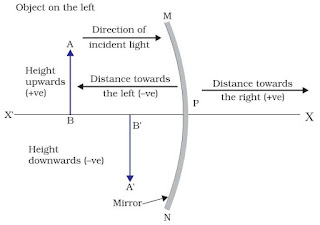• Object distance = ‘u’ is always negative.
• Focal length of concave mirror = Negative
• Focal length of convex mirror = Positive

Mirror Formula

1/v + 1/u = 1/f

where, v = Image distance
u = Object distance
f = Focal length

Magnification of Spherical Mirrors

It is the ratio of the height of image to the height of object.

m = Height of image/Height of object
⇒ m = hi/ho
Also, m = -v/u

→ If ‘m’ is negative, image is real.
→ If ‘m’ is positive, image is virtual.
→ If hi = ho then m = 1, i.e., image is equal to object.
→ If hi > ho then m > 1 i.e., image is enlarged.
→ If hi < ho then m < 1 i.e., image is diminished.

• Magnification of plane mirror is always + 1.